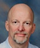# Point of Total Assumption

The point of total assumption (PTA) is a price determined by a fixed price plus incentive fee contract (FPIF) above which the seller bears all the loss of a cost overrun. It is also known as the "most pessimistic cost" because it represents the highest point beyond which costs are not expected to rise, given reasonable issues. If costs go beyond the PTA, they are assumed to be due to mis-management rather than a worst-case set of difficulties. The seller bears all of the cost risk at PTA and beyond. In addition, once the costs on an FPIF contract reach PTA, the maximum amount the buyer will pay is the ceiling price.

Any FPIF contract specifies a target cost, a target profit, a target price, a ceiling price, and one or more share ratios. The PTA is the difference between the ceiling and target prices, divided by the buyer's portion of the share ratio for that price range, plus the target cost.

PTA = ((Ceiling Price - Target Price)/buyer's Share Ratio) + Target Cost

Example:

Target Cost: 60,000

Target profit: 6000

Target Price: 63,000

Celing Price: 65,000

Share Ratio: 70% Buyer and 30% seller

PTA = (( 65000 - 63000 ) / 0.7 ) + 60000 = 62857

Source: Wikipedia.org

## Comments

### Point of Assumption

For cost reimbursable contract, Point of Total Assumption (also referred to as break point) is calculated by the following formula: PTA = [{(Ceiling Price - (Target Cost+Fixed fee))/Benefit Sharing} + Target Cost]

Example: target Cost = 1,000,000; Fixed Fee = 100,000; benefit/cost sharing = 80%/20%; Price ceiling = 1,200,000

PTA = {(1,200,000 - (1,000,000+100,000))/0.80}+1,000,000 = 1,125,000 Contributed by Dipanjan

The idea of a "Point of Total Assumption" is an extremely recent one, and even high-level government logistics specialists often don't know what the term means, or how to calculate it. In the government's efforts to cut cost overruns, however, the PTA is being introduced to FPIF contracts on an increasingly wide basis. Moreover, high-level professional examinations for industry certification, such as the Project Management Professional certification, are beginning to test applicants' knowledge of this concept and its application.

Source : Wikipedia.org

### Hi experts,  FPIP uses

Hi experts,

FPIP uses Buyer's Share ratio while Cost Reimbursable uses Benefit Sharing.

Are they the same meaning?

For FPIP, is it that even if its given that
Share Ratio: 70% Buyer and 30% seller

we Always only pluck in the buyer ratio of 70% ?

For Cost Reimbursable, if its given that

benefit/cost sharing = 80%/20% , which is the buyer's and which is the seller's ratio?

or is it that if there is a profit, the ratio to pluck in the formula is 80% while if there is a loss, the ratio to pluck in is 20% ?

Really hope to get clarification on the above doubts.

regards

J### More to PTA than shown here

The point of total assumption is not a "recent development" as it has been in use, and studied, by government and military personnel for a long time. However, it is a hot topic among PMP applicants because it recently (approximately 2007) appeared on the PMP certification exam.

Interested persons may visit www.trainingdhughes.com and click resources to find a carefully researched article which explains the concept and clarifies misunderstandings.

### More on Point of Total Assumption (PTA)

Here's another article that you might find useful on PTA:

Interesting Facts about Point of Total Assumption (PTA)

### Admin, it seems there is a

Admin, it seems there is a mistake in your example.

Target Price = Target Cost + Target Profit

So Target Profit here should be 3000, instead of 6000.

Cheers

### GRAPHICAL PRESENTATION OF PTA

Hi

A GRAPHICAL VIEW OF PTA MAKES IT EASY TO UNDERSTAND, ITS FORMULA PROOFALSO CAN BE DERIVED BY THE GRAPH.

SEE AT MY BLOG:

http://er-sspawar.blogspot.in/

POSTING 2, CLICK ON FIGURE YOU WILL GET INLARGED AND UNDERSTABLE VIEW.

REGARDS

### Points of Total Assumption

Hi,

Target price is Sum(Target cost +Target Profit). So shouldnot target price be 66000 instead of mentioned Target Price of 63000 in the above question?

Please reply.

### writing a newsletter

<a href="http://www.newsletterwritingservices.net/how-we-are-writing-a-newsletter/">writing a newsletter</a>
Gær gjaldeyrismarkaðir evru laust sögulegt þak 60 rúblur. Í morgun viðskipti á Moskvu Kauphöllinni til að opna námskeiðið 62,031₽ / €, 30 cent ofan loka gær. Dollar viðskipti á 50 rúblur hefur ekki vaxið, en markaðsaðilar fastur blettur gjaldmiðil kaup- og spyrja verð á stigi ofan.

### PTA

I wanted to know how to calculate PTA.Thanks for tips.